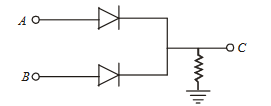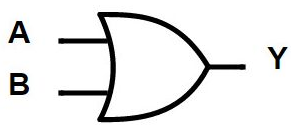Q

# Solve! In the circuit below, A and B represent two inputs and C represents the output. The circuit represents

In the circuit below, A and B  represent two inputs and represents the output. The circuit represents• Option 1)

OR gate

• Option 2)

NOR gate

• Option 3)

AND gate

• Option 4)

NAND gate.

1172 Views

As we learnt in

OR gate -

Relation between input and output- wherein

A and B are input

Y is out put

This circuit represents OR gate.

Since when either of the two terminal are at higher potential, we get output as higher potential.

Correct option is 1.

Option 1)

OR gate

This is the correct option.

Option 2)

NOR gate

This is an incorrect option.

Option 3)

AND gate

This is an incorrect option.

Option 4)

NAND gate.

This is an incorrect option.

Exams
Articles
Questions